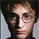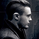16532浏览
This simple indicator gives you a lot of useful information - when to enter, when to exit
and how to reduce risks by entering a trade on a double confirmed signal.
You can use in the xPrice any series: Open, High, Low, Close, HL2 , HLC3, OHLC4 and ect...
```////////////////////////////////////////////////////////////
//  Copyright by HPotter v1.0 25/07/2014
// This simple indicator gives you a lot of useful information - when to enter, when to exit
// and how to reduce risks by entering a trade on a double confirmed signal.
// You can use in the xPrice any series: Open, High, Low, Close, HL2, HLC3, OHLC4 and ect...
////////////////////////////////////////////////////////////
study(title="FX Sniper:  T3-CCI Strategy", shorttitle="T3-CCI")
CCI_Period = input(14, minval=1)
T3_Period = input(5, minval=1)
b = input(0.618)
hline(0, color=purple, linestyle=line)
xPrice = close
b2 = b*b
b3 = b2*b
c1 = -b3
c2 = (3*(b2 + b3))
c3 = -3*(2*b2 + b + b3)
c4 = (1 + 3*b + b3 + 3*b2)
nn = iff(T3_Period < 1, 1, T3_Period)
nr = 1 + 0.5*(nn - 1)
w1 = 2 / (nr + 1)
w2 = 1 - w1
xcci = cci(xPrice, CCI_Period)
e1 = w1*xcci + w2*nz(e1)
e2 = w1*e1 + w2*nz(e2)
e3 = w1*e2 + w2*nz(e3)
e4 = w1*e3 + w2*nz(e4)
e5 = w1*e4 + w2*nz(e5)
e6 = w1*e5 + w2*nz(e6)
xccir = c1*e6 + c2*e5 + c3*e4 + c4*e3
cciHcolor = iff(xccir >= 0 , green,
iff(xccir < 0, red, black))
pos = iff(xccir > 0, 1,
iff(xccir < 0, -1, nz(pos, 0)))
barcolor(pos == -1 ? red: pos == 1 ? green : blue )
plot(xccir, color=blue, title="T3-CCI")
plot(xccir, color=cciHcolor, title="CCIH", style = histogram)```

## 评论Lucaaa
@Lucaaa, Do not know. It should be tested for each tf and symbol.Hi HPotter,
First of all thanks for the strategy, it is really nice and useful.
I wanted to ask you if you have the same issue I'm having: I sometimes get double alerts on different candles. So, for example, if I entered short, maybe after a candle or two the wave would again "touch" the trend line and consider itself as a crossing down, triggering a second alert.

Is there any way to avoid this?

Thanks!gxyzod
@gxyzod, It may be problem to work realtime. If you try to set xPrice = close it can be fix it.Good afternoon! Thanks for the great indicator! Is it possible to create alerts when changing a trend? Thank you!Sorry @HPotter , I have tried to hear your advices but i didn't manage to set the indicator because i'' don't understand how to set it ...could you help me please? Thanks in advanceEmisfero
You should add to favorite and then it should be in the Indicators list as a Favorite